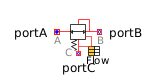Matrix Gain - MapleSim Help

Matrix Gain

Matrix ProductDescription The Matrix Gain component computes the product of a gain matrix, K, with the input vector $u$. To change the dimensions of the gain matrix, right-click (Control-click for Mac) the K parameter field and select Edit Matrix Dimensions. You can then specify the number of rows and columns to include in the matrix. Note: The context menu operation for setting the matrix dimension is not available if the parameter field is blank. In this case, you must enter a matrix as a list of lists, for example, [[1,2],[3,4]].Equations $y=K\cdot u$Connections

 Name Description Modelica ID $u$ Real input vector u $y$ Real output vector yParameters

 Name Default Units Description Modelica ID K $\left[\left[1,0\right],\left[0,1\right]\right]$ Gain matrix which is multiplied with the input KModelica Standard Library The component described in this topic is from the Modelica Standard Library. To view the original documentation, which includes author and copyright information, click here.